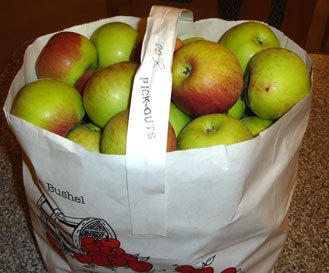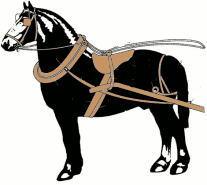WORKSHEET:ALGEBRA 3 Worksheets Descargar PDF 1) Frank and Lea buy 11 books together . Lea buys 5 books. How many books does Frank buy? Solution 2) If 4 is subtracted from twice a number, the result is 10 less than the number. Find the number. Solution 3) Three times a number is added to the number and the answer is 60. Find the number. Solution 4) Two consecutive numbers have a sum of 43. What are the numbers? Solution 5) A kilogram of cherries costs twice as much a kilogramme of apples. If I have paid 15 for three kilos of cherries and four kilos of apples. How much is a kilogramme of apples? How much is a kilogramme of cherries?Solution 6) An orange has 65 calories. This is 10 calories less than one third of the calories in an ice cream. How many calories are in an ice cream?Solution 7) The perimeter of a swimming pool is 98m. The width is 11 m less than the length. Find the width and the length of the pool. Solution 8) Fred has three bags of apples and six more apples in his backpack. Each bag contains the same number of apples. Altogether, Fred has 45 apples. How many apples are in each bag?Solution 9) A man buys a saddle, a horse, and a harness, for 360. The saddle costs twice as much as the harness ; and the horse costs twice as much as the harness and saddle together. What is the price of each?Solution 10) Jenny and Bob have a combined weight of 78 kilograms. Jenny weighs 51 kilos less than twice Bobs weight. How much does each one weigh? Solution